Monotonic transformations occur frequently in math and statistics. Analysts use monotonic transformations to transform variable values, with Tukey's ladder of transformations and the Box-Cox transformations being familiar examples. Monotonic distributions figure prominently in probability theory because the cumulative distribution is a monotonic increasing function. For a continuous distribution that is strictly monotonic, the quantile function is also monotonic.

In a recent project, I needed to determine whether a certain function is monotonic increasing. Because the function is only known at a finite sequence of values, the reduced problem is to determine whether a sequence is increasing. I have previously shown that you can use the DIF function in SAS to test for an increasing sequence. This article shows how to apply the method to the problem of deciding whether a function is increasing.

### What is a monotone sequence?

There are two types of monotonicity. In a weakly monotonic sequence, adjacent terms can be equal. That is, the difference between adjacent terms is allowed to be 0. In a strongly monotonic sequence (also called strictly monotonic), adjacent terms cannot be equal. For a strictly increasing sequence, the difference between adjacent terms is always positive. For a strictly decreasing sequence, the difference between adjacent terms is always negative. By itself, the term monotonic means the sequence is either increasing or decreasing.

Thus, there are four different tests for monotonicity. You can test whether a sequence is weakly increasing, strictly increasing, weakly decreasing, or strictly decreasing.

### Test for monotone increasing in SAS

The following SAS/IML module evaluates a vector and tests whether the elements are increasing. It uses the DIF function to produce a vector that contains the difference between adjacent elements of the input vector. That is, if x = {x1, x2, x3, ..., xn} is the input vector, then d = diff(x,1,1) is the vector {x2-x1, x3-x2, ..., xn-x[n-1]}. The second argument specifies the lag. The third argument (which is available in SAS 9.4M5) is a flag that specifies whether the result is padded with missing values. (See the Appendix for more information.) By default, the function tests for a weakly increasing sequence.

```proc iml; /* Test whether a sequence of elements is monotonic increasing. Valid options are strict=0 : (Default) Return 1 if a sequence is nondecreasing strict=1 : Return 1 if a sequence is strictly increasing */ start IsIncr(_x, strict=0); x = colvec(_x); if nrow(x)=1 then return(1); /* one element is always monotonic! */ d = dif(x,1,1); /* lag=1; delete initial missing value */ if strict then return( all(d > 0) ); return( all(d >= 0) ); finish;   /* test whether sequences are increasing */ x = {0,2,2,2,6,7,9}; y = {0,1,3,4,6,7,9}; z = {0,1,3,4,2,7,9}; b1 = IsIncr(x); /* test weakly increasing */ b2 = IsIncr(x, 1); /* test strictly increasing */ b3 = IsIncr(y, 1); /* test strictly increasing */ b4 = IsIncr(z); /* test weakly increasing */   print b1 b2 b3 b4;```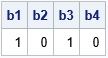The IsIncr function is called four times:

1. The first call returns the value 1 because the elements of x are weakly monotonic (nondecreasing).
2. The second call returns the value 0 because the elements of x are not strictly increasing.
3. The third call returns the value 1 because the elements of y are strictly increasing.
4. The fourth call returns the value 0 because the elements of z are not monotonic.

### Test for monotone decreasing in SAS

If a sequence {x1, x2, x3, ...} is monotone increasing, then the sequence obtained by multiplying each element by -1 is monotone decreasing. Therefore, it is trivial to write a function that tests whether a sequence is decreasing: simply test whether the negative of the sequence is increasing! This is accomplished by the following SAS/IML function:

```/* Test whether a sequence of elements is monotonic decreasing. strict=0 : (Default) Return 1 if a sequence is nonincreasing strict=1 : Return 1 if a sequence is strictly decreasing */ start IsDecr(x, strict=0); return IsIncr(-x, strict); finish;   /* test whether sequence is increasing */ u = {9,8,7,7,6,2,0}; b5 = IsDecr(u); /* test weakly decreasing */ b6 = IsDecr(u, 1); /* test strictly decreasing */ print b5 b6;```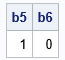The sequence is weakly decreasing but not strictly decreasing. The first call to the IsDecr function returns 1. The second call returns 0.

### An application: Test whether a transformation is monotonic

I used the IsIncr function to address a general question: Can you detect whether an unknown univariate function is monotonic increasing?

In a recent project, I was modeling the cumulative distribution function (CDF) of an unknown continuous distribution. By definition, a CDF must be increasing, but for some values of the parameters, the model is not an increasing function. I needed to identify these "infeasible" parameter values in a quick and reliable manner.

Mathematically, an increasing function, F, has the property that for every increasing sequence, {x_i}, the image of that sequence, {F(x_i)}, is also increasing. Numerically, you can generate an increasing sequence in the domain and ask whether the image of that sequence is also increasing.

Here's an example. Suppose you want to test whether the following functions are increasing:
F1(x) = (5 - 4*x) log( x/(1-x) )
F2(x) = (5 - 5.2*x) log( x/(1-x) )
The functions are defined on the domain (0, 1). The following program defines an increasing sequence on (0,1) and tests whether the image of the sequence is also increasing for F1 and for F2:

```start Func1(x); return (5 - 4*x)#log( x/(1-x) ); finish; start Func2(x); return (5 - 5.2*x)#log( x/(1-x) ); finish;   dt = 0.005; x = do(dt, 1-dt, dt); /* increasing sequence on a fine grid */ y1 = Func1(x); /* image of sequence under F1 */ y2 = Func2(x); /* image of sequence under F2 */ b1 = IsIncr(y1); b2 = IsIncr(y2); print b1 b2;```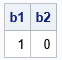The output indicates that the first function in increasing, but the second function is not. The following graph of the second function shows, indeed, that the function is not strictly increasing.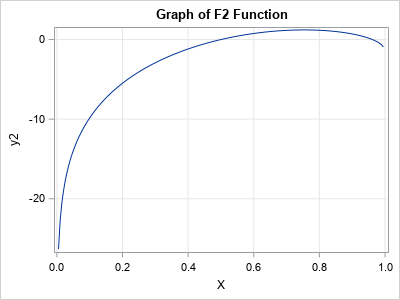The accuracy of this method depends on choosing a sequence on a fine grid of points in the domain. It assumes that the derivative of the function is bounded so that function cannot quickly increase and decrease on one of the small gaps between consecutive elements of the sequence.

### Summary

This article shows a way to test whether a function is monotonic on an interval. A common application is to test for an increasing function, but it is equally easy to test for a decreasing function. You can test whether the function is weakly increasing or strictly increasing.

These numerical tests evaluate a function at a finite set of points. Consequently, they can rule out the hypothesis of monotonicity, but they do not prove that the function is increasing everywhere. Nevertheless, these tests work well in practice if you choose the input sequence on a fine grid in the domain. If you have a mathematical bound on the derivative of the function, you can say more.

### Appendix: A useful option for the DIF function

By default, the DIF function in SAS/IML returns a vector that is the same size as the input vector. If k is the lag parameter, the first k elements are missing values. The differences between elements are contained in the elements k+1, k+2, and so forth. If you specify, the value 1 for the third option, the DIF function deletes the leading missing values. The result is a vector that has n – k elements, where n is the length of the input vector and k is the lag parameter.

For example, the following example passes in k=1 and deletes the leading missing value for the result:

```t = {0,3,3,2,7}; /* input argument has 5 elements */ d = dif(t, 1, 1); /* LAG=1; delete leading missing value */ print d; /* result has 4 elements */```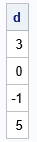Share